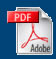Home English Maths Science ICT History Geography Art Year Six Revision Maths APP
Home > Mathematics > Datahandling

Datahandling

Key Objectives here are to be able to use Carroll Diagrams and Venn Diagrams effectively.Carroll DiagramsTally ListDrawing a graphVenn Diagrams 1Venn Diagrams 2Venn Diagrams 3Venn Diagrams 4Tally charts and block chartsTally Chart DataVenn Diagrams 5Venn Diagrams 6Venn Diagrams 7Pictograms (Harder)Pictograms (Easier)Tables 1Tables 2House InvestigationHouse Information SheetInteractive Carroll DiagramsCarroll and Venn Diagrams 1Carroll and Venn Diagram 2Bar Chart Prompt SheetHistograms [Vid 140]Histograms Information (Visio)Pictogram 1Pictogram 2Fruit PictogramsVenn Diagram Story for Edwards Venn Diagrams

Some History for Data Handling

There are lots of technical terms you might need to be able to use to do with this part of maths. It is associated with a part of maths called statistics. Benjamin D'israeli, a famous Prime Minister, once said, "There are three types of lies: Lies, damned lies and statistics." He said this because you can often use statistics to put forward your ideas and people will assume they are right because of the power of numbers. The moral is: be careful when people start quoting statistics at you.

A major advocate of statistics was Florence Nightingale. She actually invented the pie chart in order to persuade the government of the importance of health care and medical facilities.

Did you know that Venn Diagrams were first thought of by John Venn in July 1880. Carroll diagrams were invented by Lewis Carroll who also wrote Alice in Wonderland.

Technical Vocabulary for Data Handling

Statistics - the study of data.

There are two main types of data: discrete and continuous.

Discrete data is counted data (when we do a survey of favourite things).

Continuous data is measured or calculated data (when we measure the temperature).

We will be mainly looking at discrete data in this unit. (Remember: discrete data is counted)

 Continuous Data Discrete Data Measured or calculated Counted Graphs Charts No gaps in graphs Gaps between bars in charts.

A chart is a data display for discrete data which has been counted.

A graph is a data display for continuous data which has been calculated or measured.

Sort means putting things into order.

Select means choosing specific things.

Maximum means the most of something.

Minimum means the least of something.

There are three types of arithmetic average. That is why you never hear a mathematician say, "The average is..." because it isn't clear what is meant. You will often hear politicians say, "The average is..." for very same reason.

The mean average is the one most people mean, most of the time. Add up all the numbers and then divide by the number of numbers.

The median average is the one that is in the middle. Put the numbers into order and then find the middle one.

The mode is the one that is the most common. Which thing occurs most often? That is the mode.

The range is the difference between the maximum and the minimum values.

There is a range called the Interquartile range. To work this out, you need to put all the values in order. Find the median value and then find the median value of the lower half of the numbers and then the median value of the upper half of the numbers. This should give you a value that is one quarter of the way up the data and three quarters of the way up the data. The difference between these two values is the Interquartile range. (You don't need this until you are doing GCSE, so don't worry about it).

Logic is about organising and sorting information. Venn Diagrams and Carroll Diagrams are two types of logic tool.